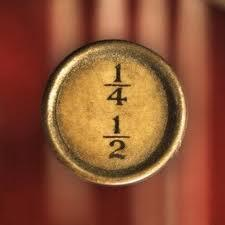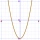# Factors

Can the expression
$4{x}^{2}-47.6x+39.6$
be factored into rational factors?

Result

D =  1632.16

#### Solution:

D= 40.4^2

D = 1632.16

D = 4080425 = 1632.16

Our simple equation calculator calculates it.We would be pleased if you find an error in the word problem, spelling mistakes, or inaccuracies and send it to us. Thank you!Tips to related online calculators

## Next similar math problems:

• DiscriminantDetermine the discriminant of the equation: ?
• Geometric progression 48,4√2,4,2√2
• Is complexAre these numbers 2i, 4i, 2i + 1, 8i, 2i + 3, 4 + 7i, 8i, 8i + 4, 5i, 6i, 3i complex?Which of the points belong function f:y= 2x2- 3x + 1 : A(-2, 15) B (3,10) C (1,4)
• CalculationHow much is sum of square root of six and the square root of 225?
• Solve 3Solve quadratic equation: (6n+1) (4n-1) = 3n2
• Find the 20Find the product and the sum of the roots of x2 + 3x - 9 = 0Find the roots of the quadratic equation: 3x2-4x + (-4) = 0.
• CombinationsFrom how many elements we can create 990 combinations 2nd class without repeating?Solve quadratic equation: 2x2-58x+396=0
• Variations 4/2Determine the number of items when the count of variations of fourth class without repeating is 600 times larger than the count of variations of second class without repetition.
• EquationEquation ? has one root x1 = 8. Determine the coefficient b and the second root x2.
• ProductThe product of two consecutive odd numbers is 8463. What are this numbers?
• RootsDetermine the quadratic equation absolute coefficient q, that the equation has a real double root and the root x calculate: ?Quadratic equation ? has roots x1 = -26 and x2 = -86. Calculate the coefficients b and c.F(x)=x2-7x and g(x)=5-x2 Domain of (fg)(x) is. .. . . Domain of (f/g)(x). ..Solve the system of non-linear equations: 3x2-3x-y=-2 -6x2-x-y=-7